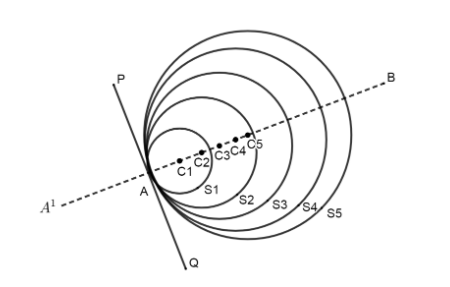Courses
Courses for Kids
Free study material
Free LIVE classes
MoreLIVE
Join Vedantu’s FREE Mastercalss

# Write true or false and justify your answer in each of the following:If a number of circles touch a given line segment PQ at a point A, then their centers lie on the perpendicular bisector of PQ.(A) True(B) False(C) Ambiguous(D) Data insufficientVerified
333.9k+ views
Hint: To solve this question, we will draw a line and we will draw a number of circles touching that line. After doing this, we will find the focus of the centers of all these circles and then we will answer according to the options given in question

Now, in the question, it is given that a number of circles touch the given line segment at a point. So, we will draw the figure as the information given in question:Here, in the above figure, we have drawn five circles ${{\text{S}}_{1}},\text{ }{{\text{S}}_{2}},\text{ }{{\text{S}}_{3}},\text{ }{{\text{S}}_{4}}\text{ and }{{\text{S}}_{5}}$ which has centers ${{\text{C}}_{1}},\text{ }{{\text{C}}_{2}},\text{ }{{\text{C}}_{3}},\text{ }{{\text{C}}_{4}}\text{ and }{{\text{C}}_{5}}$ the point at which all these circles touch the line segment PQ is A. we can also see from the figure that the centers of circles lie on a straight line. Now, we have to determine the nature of this line. We know that if a line touches a circle at only one point then that line will be the tangent of the circle at that point. In our question, it is given that all the circles touch the line segment PQ at A. Thus the line PQ will be the tangent to all these circles. Now, the line AB passes through the centers of all these circles. It also passes through the point A. Thus the line AB will be normal to all these circles. Thus, we can say that the line ${{\text{A}}^{1}}\text{B}$will be perpendicular to line PQ. But here, we do not know anything about the point A i.e. at which position does it lie in the line segment.
Hence, option (D) is correct, because we are not given any information about the point A. If A is the midpoint of the line segment PQ then ${{\text{A}}^{1}}\text{ B}$ will be a perpendicular bisector otherwise it will be not. So the answer depends on the point on the position of A.

Note: Here, while solving the question, we have considered that the circles lie in the second plane (x-y plane). Even if there is a circle in a third plane (x-y-z plane) and it is touching a given line segment at point A, its center will be on the perpendicular bisector of PQ. Another thing to note is that the radius of the circle has no role in it. Any circle of real radius will satisfy the condition.

Last updated date: 21st Sep 2023
Total views: 333.9k
Views today: 9.33k